# GMAT Math : DSQ: Calculating whether point is on a line with an equation

## Example Questions

### Example Question #1 : Dsq: Calculating Whether Point Is On A Line With An Equation

Determine whether the points are collinear.

Statement 1: The three points are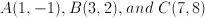Statement 2: Slope of line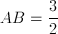and the slope of line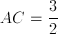Statements 1 and 2 TOGETHER are NOT sufficient.

Statement 1 ALONE is sufficient, but statement 2 is not sufficient.

Statement 2 ALONE is sufficient, but statement 1 is not sufficient.

BOTH statements TOGETHER are sufficient, but NEITHER statement ALONE is sufficient.

EACH statement ALONE is sufficient.

EACH statement ALONE is sufficient.

Explanation:

Points are collinear if they lie on the same line.  Here A, B, and C are collinear if the line AB is the same as the line AC.  In other words, the slopes of line AB and line AC must be the same.  Statement 2 gives us the two slopes, so we know that Statement 2 is sufficient.  Statement 1 also gives us all of the information we need, however, because we can easily find the slopes from the vertices.  Therefore both statements alone are sufficient.

### Example Question #2 : Dsq: Calculating Whether Point Is On A Line With An Equation

Given: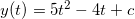Find.

I)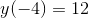.

II) Thecoordinate of the minmum of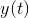is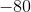.

Statement I is sufficient to answer the question, but statement II is not sufficient to answer the question.

Statement II is sufficient to answer the question, but statement I is not sufficient to answer the question.

Either statement is sufficient to answer the question.

Both statements are needed to answer the question.

Either statement is sufficient to answer the question.

Explanation:

By using I) we know that the given point is on the line of the equation.

So I) is sufficient.

II) gives us the y coordinate of the minimum. In a quadratic equation, this is what  "c" represents.

Therefore, c=-80 and II) is also sufficient.

### Example Question #3 : Dsq: Calculating Whether Point Is On A Line With An Equation

Find whether the point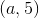is on the line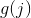.

I)is modeled by the following: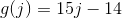.

II)is equal to five more than 3 times the y-intercept of.

Statement I is sufficient to answer the question, but Statement II is not sufficient to answer the question.

Either statement is sufficient to answer the question.

Statement II is sufficient to answer the question, but Statement I is not sufficient to answer the question.

Both statements are needed to answer the question.

Both statements are needed to answer the question.

Explanation:

To find out if a point is on line with an equation, one can simply plug in the point; however, this is complicated here by the fact that we are missing the x-coordinate.

Statement I gives us our function.

Statement II gives us a clue to find the value of.is five more than 3 times the y-intercept of. So, we can find the following: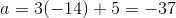To see if the point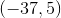is on the line, plug it into the function: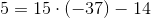This is not a true statement, so the point is not on the line.

### Example Question #4 : Dsq: Calculating Whether Point Is On A Line With An Equation

Consider linear functions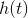andI)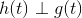at the point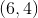.

II)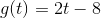Is the point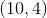on the line?

Both statements are needed to answer the question.

Statement I is sufficient to answer the question, but statement II is not sufficient to answer the question.

Statement II is sufficient to answer the question, but statement I is not sufficient to answer the question.

Either statement is sufficient to answer the question.

Both statements are needed to answer the question.

Explanation:

Consider linear functions h(t) and g(t).

I)at the pointII)Is the pointon the line h(t)?

We can use II) and I) to find the slope of h(t)

Recall that perpendicular lines have opposite reciprocal slope. Thus, the slope of h(t) must be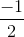Next, we know that h(t) must pass through (6,4), so lets us that to find the y-intercept: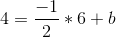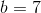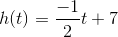Next, check if (10,4) is on h(t) by plugging it in.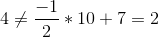So, the point is not on the line, and we needed both statements to know.

### Example Question #5 : Dsq: Calculating Whether Point Is On A Line With An Equation

Line m is perpendicular to the line l which is defined by the equation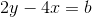. What is the value of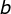?

(1) Line m passes through the point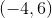.

(2) Line l passes through the point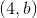.

Statements (1) and (2) TOGETHER are NOT sufficient.

Statement (2) ALONE is sufficient, but statement (1) alone is not sufficient.

Statement (1) ALONE is sufficient, but statement (2) alone is not sufficient.

EACH statement ALONE is sufficient.

BOTH statements TOGETHER are sufficient, but NEITHER statement ALONE is sufficient.

Statement (2) ALONE is sufficient, but statement (1) alone is not sufficient.

Explanation:

Statement 1 allows you to define the equation of line m, but does not provide enough information to solve for.  There are still 3 variables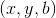and only two different equations to solve.

if, statement 2 supplies enough information to solve for b by substitution ifis on the line.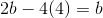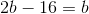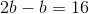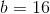Tired of practice problems?

Try live online GMAT prep today.